Скачать презентацию UNIVERSITY OF MASSACHUSETTS Dept of Electrical Computer

22cb69fd5bbbd515c69e55f8bc750ff4.ppt

• Количество слайдов: 34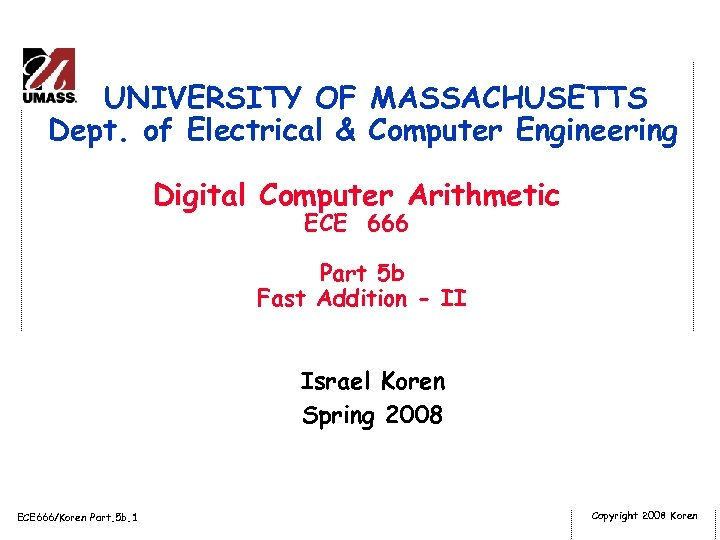UNIVERSITY OF MASSACHUSETTS Dept. of Electrical & Computer Engineering Digital Computer Arithmetic ECE 666 Part 5 b Fast Addition - II Israel Koren Spring 2008 ECE 666/Koren Part. 5 b. 1 Copyright 2008 Koren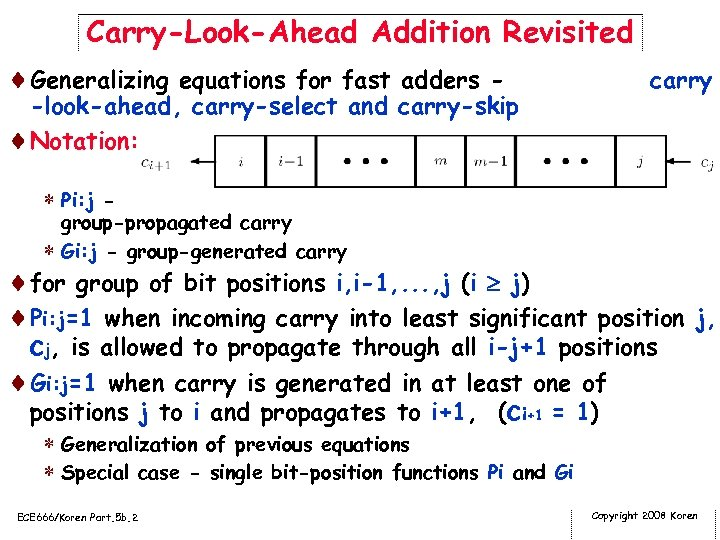Carry-Look-Ahead Addition Revisited ¨Generalizing equations for fast adders - -look-ahead, carry-select and carry-skip ¨Notation: carry * Pi: j - group-propagated carry * Gi: j - group-generated carry ¨for group of bit positions i, i-1, . . . , j (i j) ¨Pi: j=1 when incoming carry into least significant position j, cj, is allowed to propagate through all i-j+1 positions ¨Gi: j=1 when carry is generated in at least one of positions j to i and propagates to i+1, (ci+1 = 1) * Generalization of previous equations * Special case - single bit-position functions Pi and Gi ECE 666/Koren Part. 5 b. 2 Copyright 2008 Koren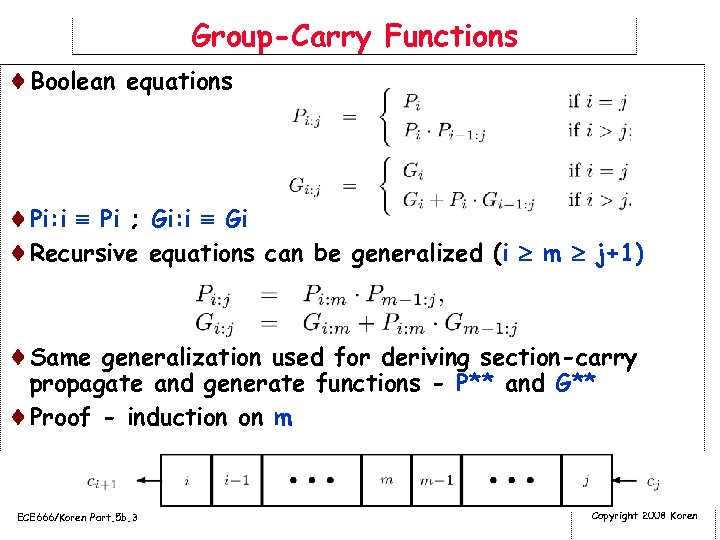Group-Carry Functions ¨Boolean equations ¨Pi: i Pi ; Gi: i Gi ¨Recursive equations can be generalized (i m j+1) ¨Same generalization used for deriving section-carry propagate and generate functions - P** and G** ¨Proof - induction on m ECE 666/Koren Part. 5 b. 3 Copyright 2008 Koren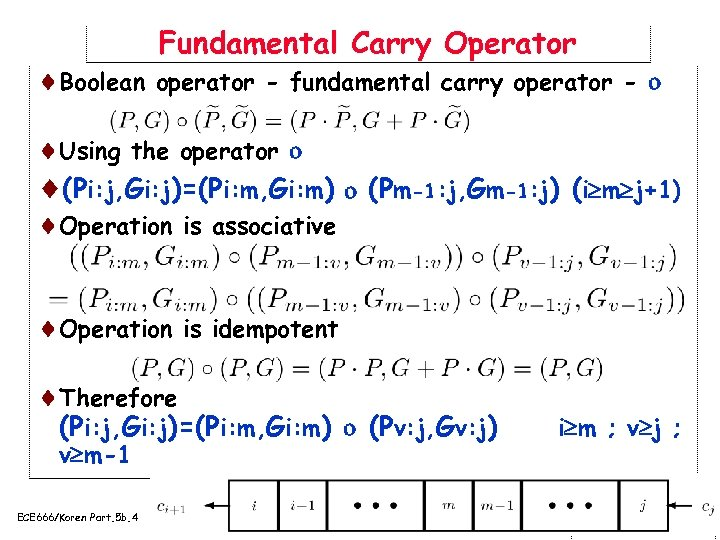Fundamental Carry Operator ¨Boolean operator - fundamental carry operator - ¨Using the operator ¨(Pi: j, Gi: j)=(Pi: m, Gi: m) (Pm-1: j, Gm-1: j) (i m j+1) ¨Operation is associative ¨Operation is idempotent ¨Therefore (Pi: j, Gi: j)=(Pi: m, Gi: m) (Pv: j, Gv: j) v m-1 ECE 666/Koren Part. 5 b. 4 i m ; v j ; Copyright 2008 Koren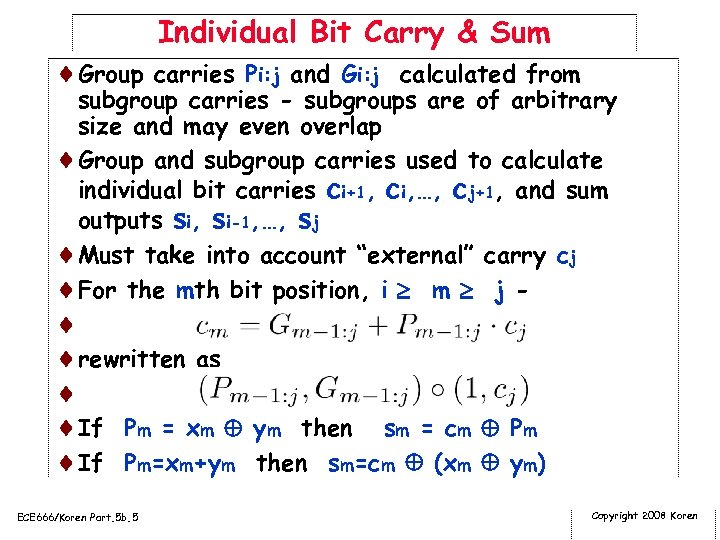Individual Bit Carry & Sum ¨Group carries Pi: j and Gi: j calculated from subgroup carries - subgroups are of arbitrary size and may even overlap ¨Group and subgroup carries used to calculate individual bit carries ci+1, ci, …, cj+1, and sum outputs si, si-1, …, sj ¨Must take into account “external” carry cj ¨For the mth bit position, i m j - ¨ ¨rewritten as ¨ ¨If Pm = xm ym then sm = cm Pm ¨If Pm=xm+ym then sm=cm (xm ym) ECE 666/Koren Part. 5 b. 5 Copyright 2008 Koren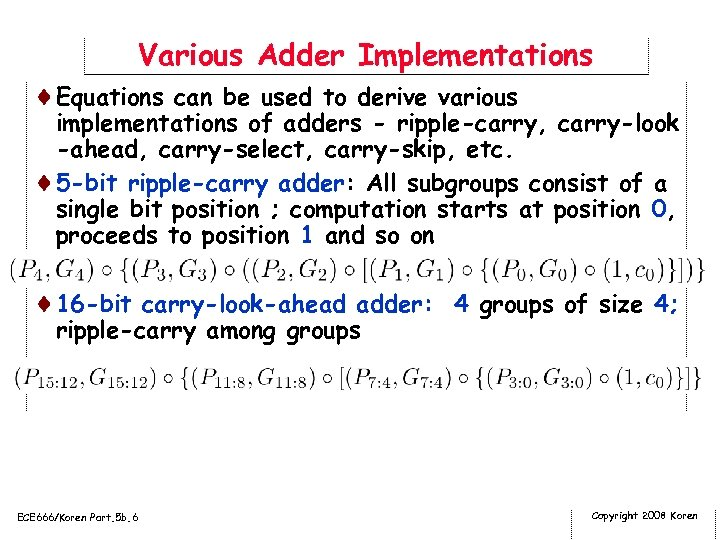Various Adder Implementations ¨Equations can be used to derive various implementations of adders - ripple-carry, carry-look -ahead, carry-select, carry-skip, etc. ¨ 5 -bit ripple-carry adder: All subgroups consist of a single bit position ; computation starts at position 0, proceeds to position 1 and so on ¨ 16 -bit carry-look-ahead adder: 4 groups of size 4; ripple-carry among groups ECE 666/Koren Part. 5 b. 6 Copyright 2008 Koren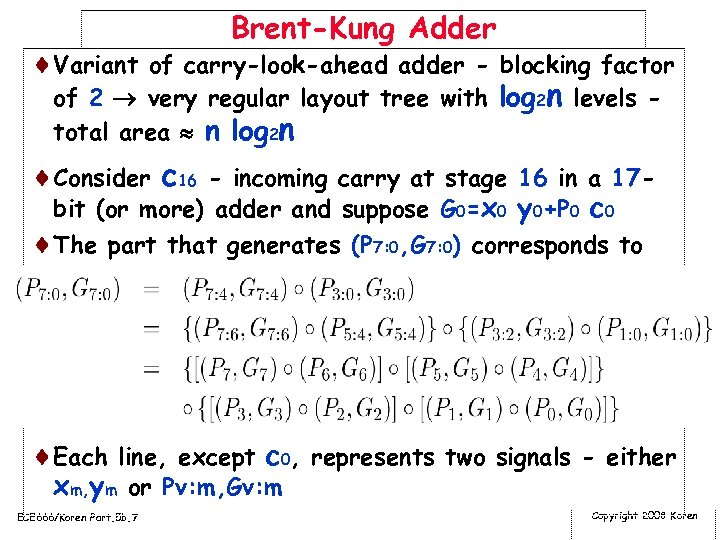Brent-Kung Adder ¨Variant of carry-look-ahead adder - blocking factor of 2 very regular layout tree with log 2 n levels total area n log 2 n ¨Consider c 16 - incoming carry at stage 16 in a 17 bit (or more) adder and suppose G 0=x 0 y 0+P 0 c 0 ¨The part that generates (P 7: 0, G 7: 0) corresponds to ¨Each line, except c 0, represents two signals - either xm, ym or Pv: m, Gv: m ECE 666/Koren Part. 5 b. 7 Copyright 2008 Koren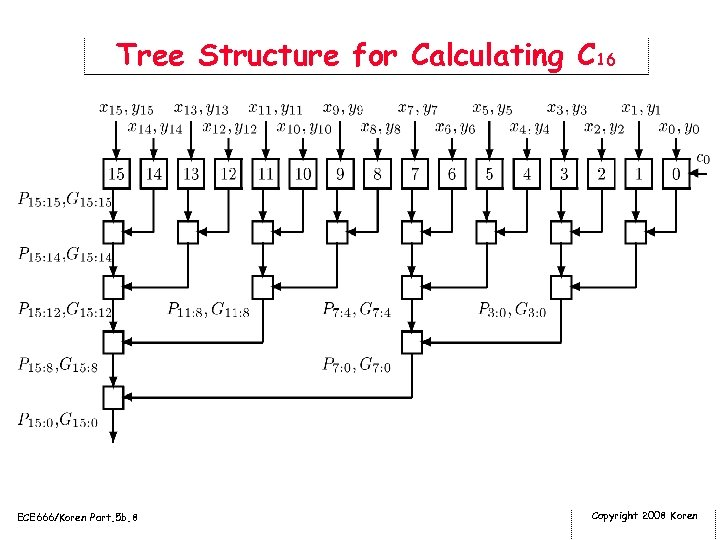Tree Structure for Calculating C 16 ECE 666/Koren Part. 5 b. 8 Copyright 2008 Koren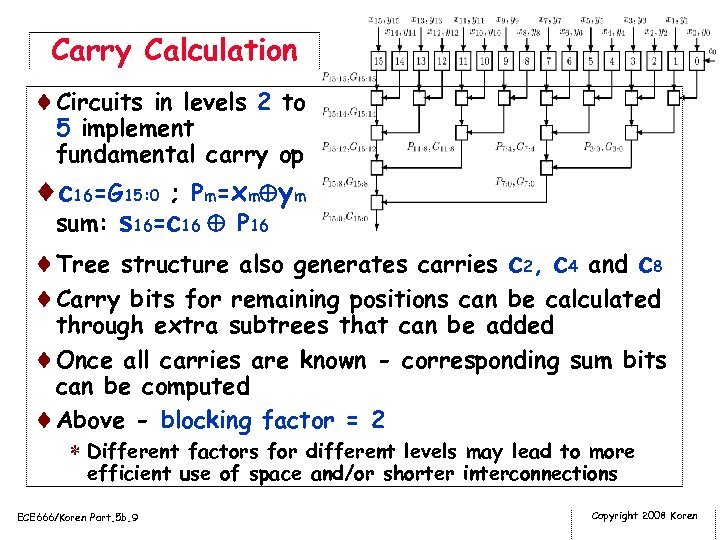Carry Calculation ¨Circuits in levels 2 to 5 implement fundamental carry op ¨c 16=G 15: 0 ; P m = x m y m sum: s 16=c 16 P 16 ¨Tree structure also generates carries c 2, c 4 and c 8 ¨Carry bits for remaining positions can be calculated through extra subtrees that can be added ¨Once all carries are known - corresponding sum bits can be computed ¨Above - blocking factor = 2 * Different factors for different levels may lead to more efficient use of space and/or shorter interconnections ECE 666/Koren Part. 5 b. 9 Copyright 2008 Koren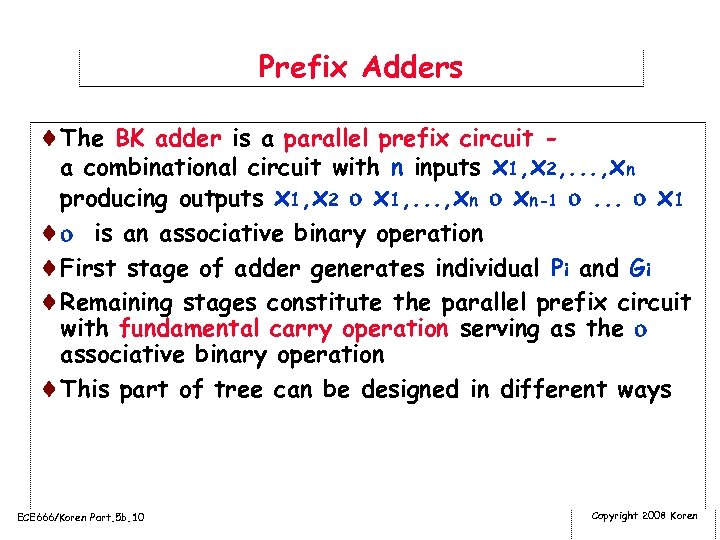Prefix Adders ¨The BK adder is a parallel prefix circuit a combinational circuit with n inputs x 1, x 2, . . . , xn producing outputs x 1, x 2 x 1, . . . , xn xn-1 . . . x 1 ¨ is an associative binary operation ¨First stage of adder generates individual Pi and Gi ¨Remaining stages constitute the parallel prefix circuit with fundamental carry operation serving as the associative binary operation ¨This part of tree can be designed in different ways ECE 666/Koren Part. 5 b. 10 Copyright 2008 Koren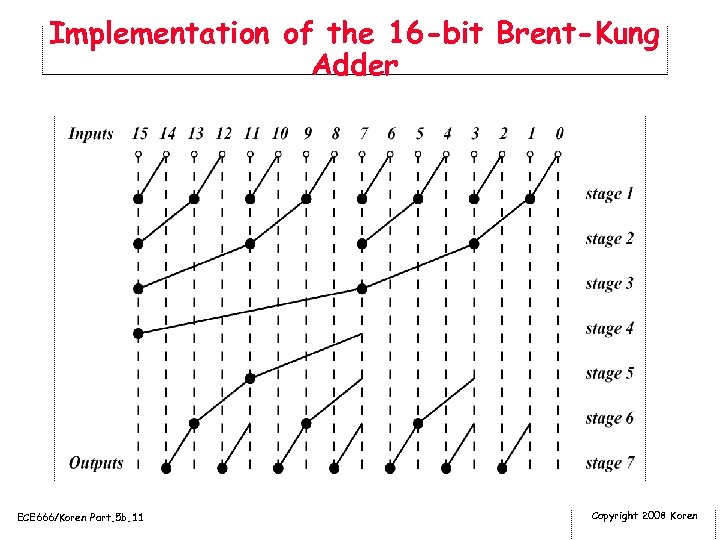Implementation of the 16 -bit Brent-Kung Adder ECE 666/Koren Part. 5 b. 11 Copyright 2008 Koren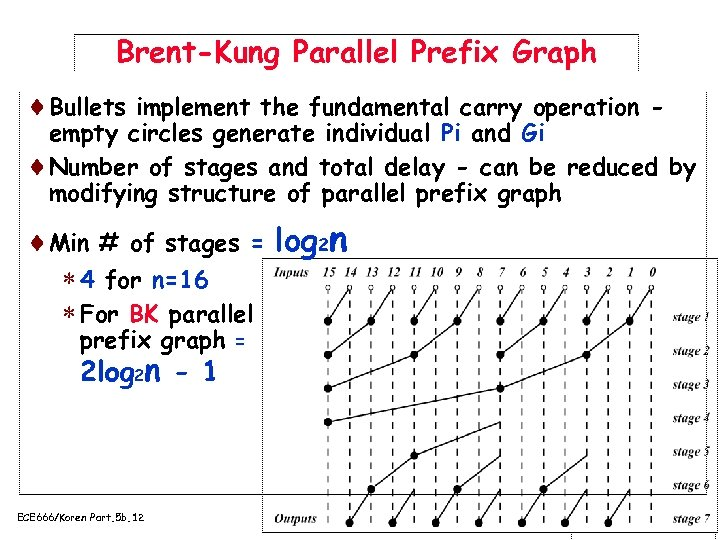Brent-Kung Parallel Prefix Graph ¨Bullets implement the fundamental carry operation - empty circles generate individual Pi and Gi ¨Number of stages and total delay - can be reduced by modifying structure of parallel prefix graph ¨Min # of stages = log 2 n * 4 for n=16 * For BK parallel prefix graph = 2 log 2 n - 1 ECE 666/Koren Part. 5 b. 12 Copyright 2008 Koren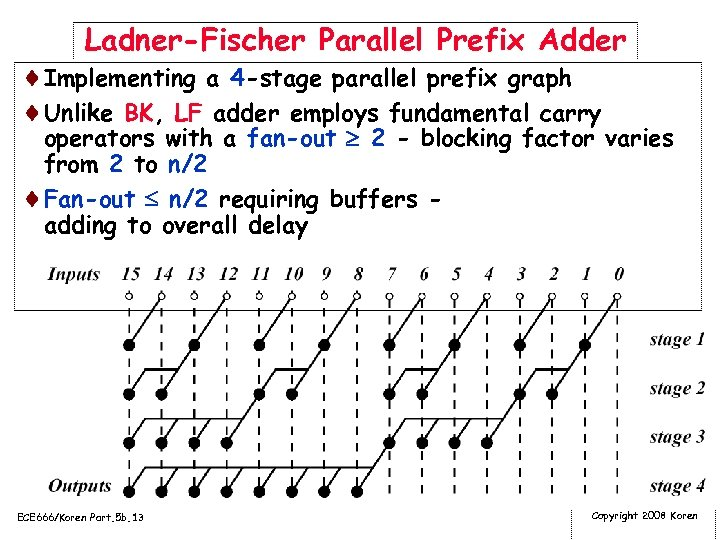Ladner-Fischer Parallel Prefix Adder ¨Implementing a 4 -stage parallel prefix graph ¨Unlike BK, LF adder employs fundamental carry operators with a fan-out 2 - blocking factor varies from 2 to n/2 ¨Fan-out n/2 requiring buffers adding to overall delay ECE 666/Koren Part. 5 b. 13 Copyright 2008 Koren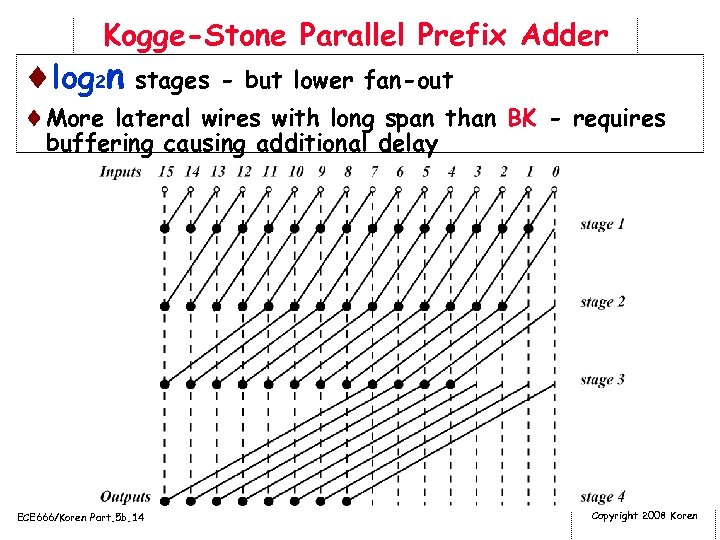Kogge-Stone Parallel Prefix Adder ¨log 2 n stages - but lower fan-out ¨More lateral wires with long span than BK - requires buffering causing additional delay ECE 666/Koren Part. 5 b. 14 Copyright 2008 Koren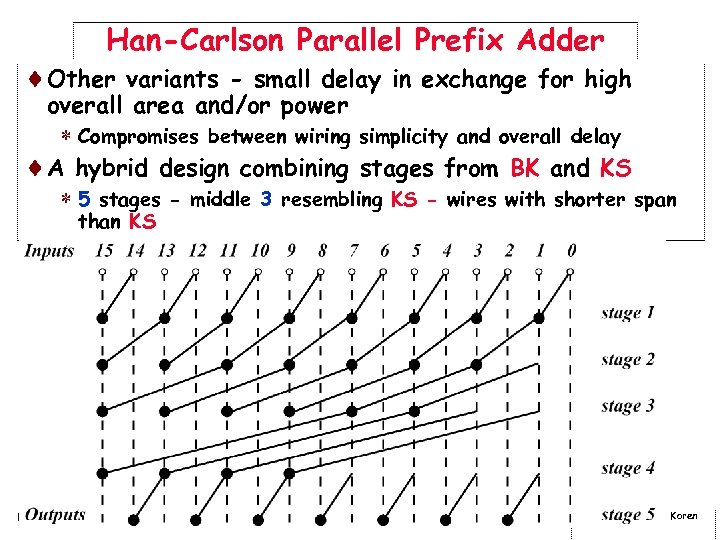Han-Carlson Parallel Prefix Adder ¨Other variants - small delay in exchange for high overall area and/or power * Compromises between wiring simplicity and overall delay ¨A hybrid design combining stages from BK and KS * 5 stages - middle 3 resembling KS - wires with shorter span than KS ECE 666/Koren Part. 5 b. 15 Copyright 2008 Koren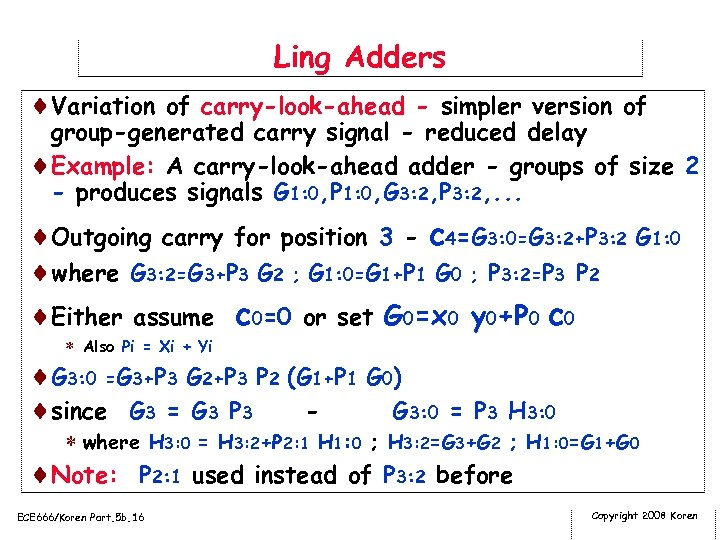Ling Adders ¨Variation of carry-look-ahead - simpler version of group-generated carry signal - reduced delay ¨Example: A carry-look-ahead adder - groups of size 2 - produces signals G 1: 0, P 1: 0, G 3: 2, P 3: 2, . . . ¨Outgoing carry for position 3 - c 4=G 3: 0=G 3: 2+P 3: 2 G 1: 0 ¨where G 3: 2=G 3+P 3 G 2 ; G 1: 0=G 1+P 1 G 0 ; P 3: 2=P 3 P 2 ¨Either assume c 0=0 or set G 0=x 0 y 0+P 0 c 0 * Also Pi = Xi + Yi ¨G 3: 0 =G 3+P 3 G 2+P 3 P 2 (G 1+P 1 G 0) ¨since G 3 = G 3 P 3 G 3: 0 = P 3 H 3: 0 * where H 3: 0 = H 3: 2+P 2: 1 H 1: 0 ; H 3: 2=G 3+G 2 ; H 1: 0=G 1+G 0 ¨Note: P 2: 1 used instead of P 3: 2 before ECE 666/Koren Part. 5 b. 16 Copyright 2008 Koren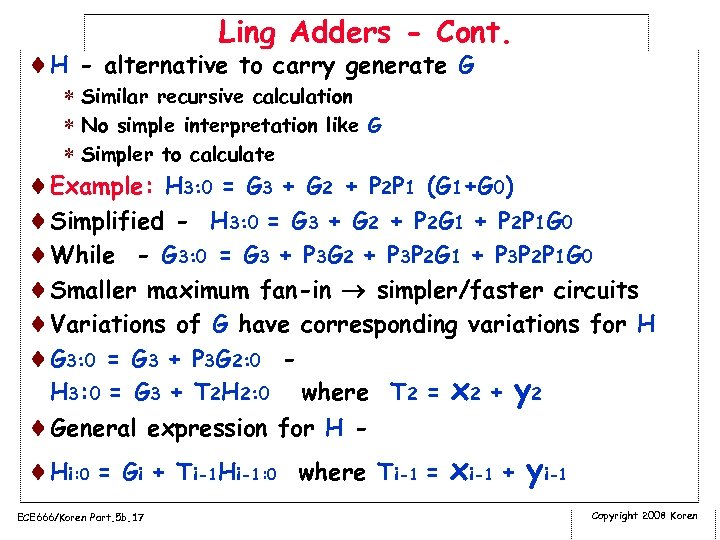Ling Adders - Cont. ¨H - alternative to carry generate G * Similar recursive calculation * No simple interpretation like G * Simpler to calculate ¨Example: H 3: 0 = G 3 + G 2 + P 2 P 1 (G 1+G 0) ¨Simplified - H 3: 0 = G 3 + G 2 + P 2 G 1 + P 2 P 1 G 0 ¨While - G 3: 0 = G 3 + P 3 G 2 + P 3 P 2 G 1 + P 3 P 2 P 1 G 0 ¨Smaller maximum fan-in simpler/faster circuits ¨Variations of G have corresponding variations for H ¨G 3: 0 = G 3 + P 3 G 2: 0 H 3: 0 = G 3 + T 2 H 2: 0 where T 2 = x 2 + y 2 ¨General expression for H - ¨Hi: 0 = Gi + Ti-1 Hi-1: 0 where Ti-1 = xi-1 + yi-1 ECE 666/Koren Part. 5 b. 17 Copyright 2008 Koren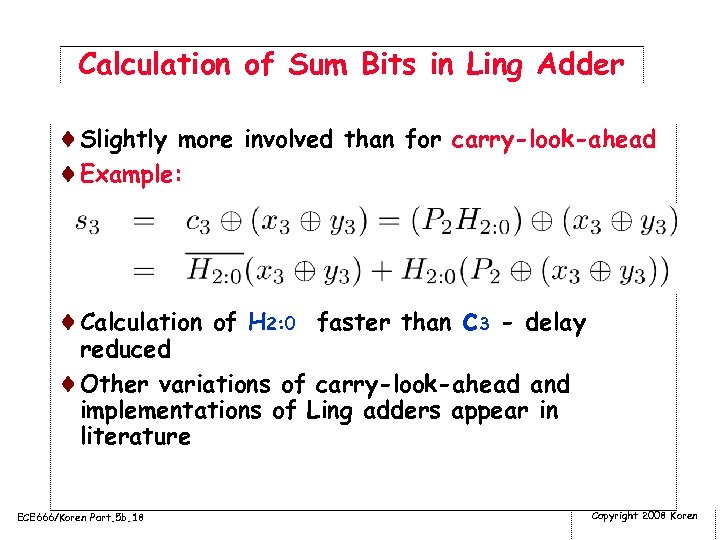Calculation of Sum Bits in Ling Adder ¨Slightly more involved than for carry-look-ahead ¨Example: ¨Calculation of H 2: 0 faster than c 3 - delay reduced ¨Other variations of carry-look-ahead and implementations of Ling adders appear in literature ECE 666/Koren Part. 5 b. 18 Copyright 2008 Koren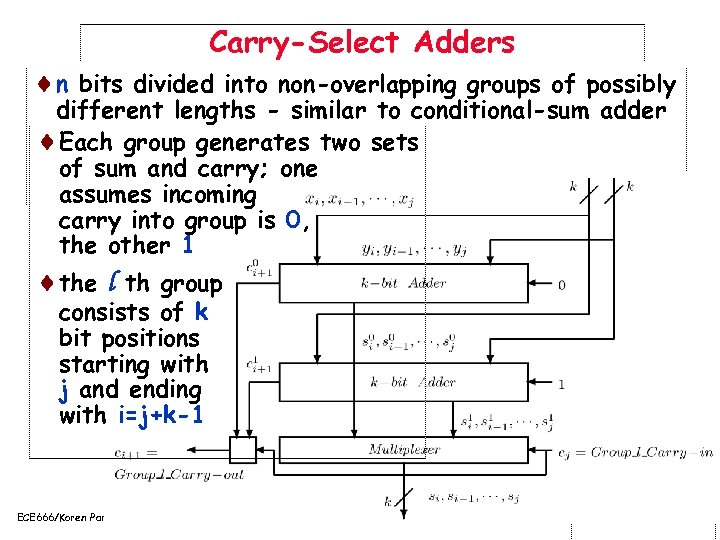Carry-Select Adders ¨n bits divided into non-overlapping groups of possibly different lengths - similar to conditional-sum adder ¨Each group generates two sets of sum and carry; one assumes incoming carry into group is 0, the other 1 ¨the l th group consists of k bit positions starting with j and ending with i=j+k-1 ECE 666/Koren Part. 5 b. 19 Copyright 2008 Koren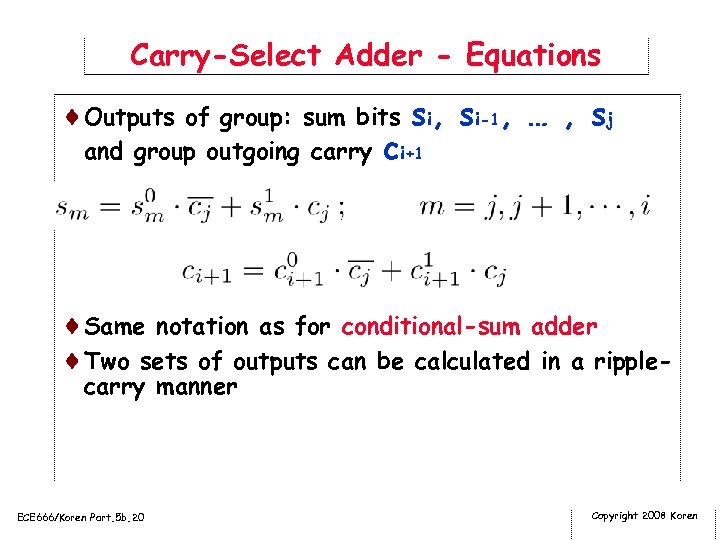Carry-Select Adder - Equations ¨Outputs of group: sum bits si, si-1, … , sj and group outgoing carry ci+1 ¨Same notation as for conditional-sum adder ¨Two sets of outputs can be calculated in a ripplecarry manner ECE 666/Koren Part. 5 b. 20 Copyright 2008 Koren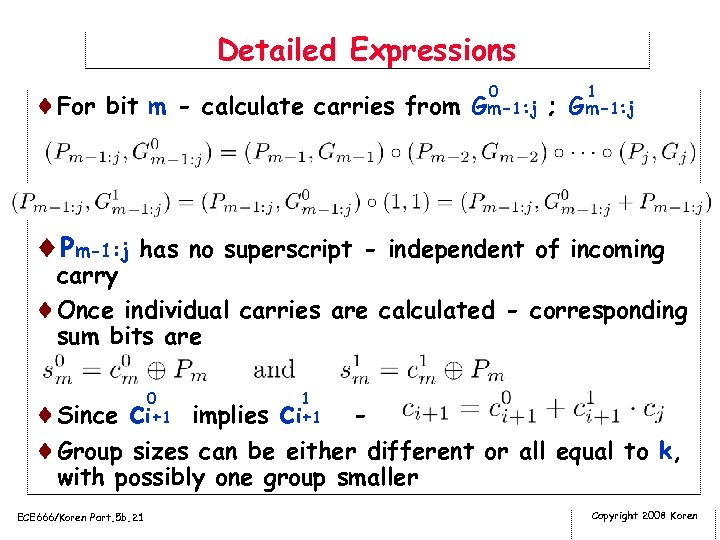Detailed Expressions 0 1 ¨For bit m - calculate carries from Gm-1: j ; Gm-1: j ¨Pm-1: j has no superscript - independent of incoming carry ¨Once individual carries are calculated - corresponding sum bits are 0 1 ¨Since ci+1 implies ci+1 ¨Group sizes can be either different or all equal to k, with possibly one group smaller ECE 666/Koren Part. 5 b. 21 Copyright 2008 Koren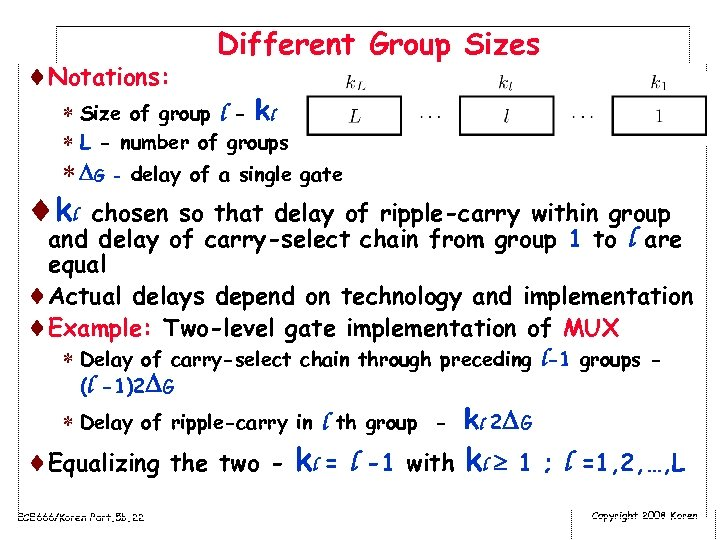¨Notations: Different Group Sizes * Size of group l - kl * L - number of groups * G - delay of a single gate ¨ kl chosen so that delay of ripple-carry within group and delay of carry-select chain from group 1 to l are equal ¨Actual delays depend on technology and implementation ¨Example: Two-level gate implementation of MUX * Delay of carry-select chain through preceding l-1 groups (l -1)2 G * Delay of ripple-carry in l th group - kl 2 G ¨Equalizing the two - kl = l -1 with kl 1 ; l =1, 2, …, L ECE 666/Koren Part. 5 b. 22 Copyright 2008 Koren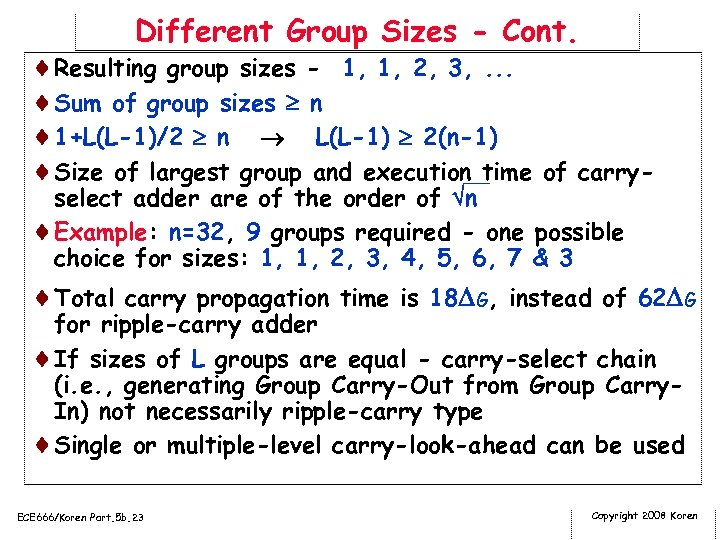Different Group Sizes - Cont. ¨Resulting group sizes - 1, 1, 2, 3, . . . ¨Sum of group sizes n ¨ 1+L(L-1)/2 n L(L-1) 2(n-1) ¨Size of largest group and execution time of carry___ select adder are of the order of n ¨Example: n=32, 9 groups required - one possible choice for sizes: 1, 1, 2, 3, 4, 5, 6, 7 & 3 ¨Total carry propagation time is 18 G, instead of 62 G for ripple-carry adder ¨If sizes of L groups are equal - carry-select chain (i. e. , generating Group Carry-Out from Group Carry. In) not necessarily ripple-carry type ¨Single or multiple-level carry-look-ahead can be used ECE 666/Koren Part. 5 b. 23 Copyright 2008 Koren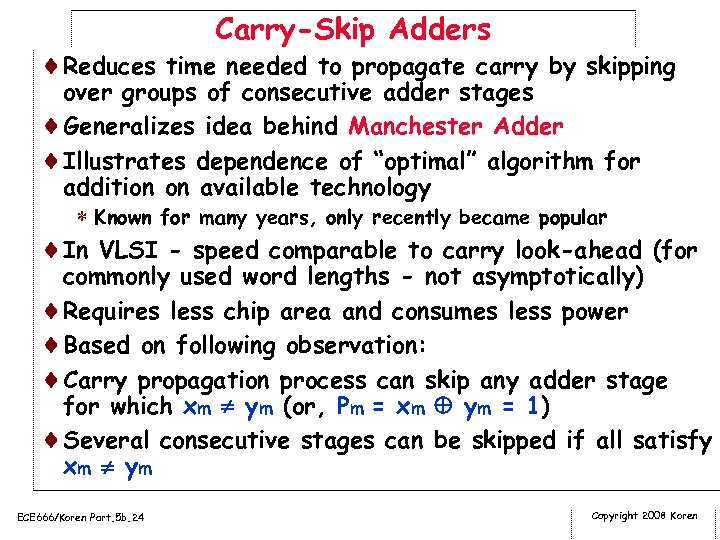Carry-Skip Adders ¨Reduces time needed to propagate carry by skipping over groups of consecutive adder stages ¨Generalizes idea behind Manchester Adder ¨Illustrates dependence of “optimal” algorithm for addition on available technology * Known for many years, only recently became popular ¨In VLSI - speed comparable to carry look-ahead (for commonly used word lengths - not asymptotically) ¨Requires less chip area and consumes less power ¨Based on following observation: ¨Carry propagation process can skip any adder stage for which xm ym (or, Pm = xm ym = 1) ¨Several consecutive stages can be skipped if all satisfy xm y m ECE 666/Koren Part. 5 b. 24 Copyright 2008 Koren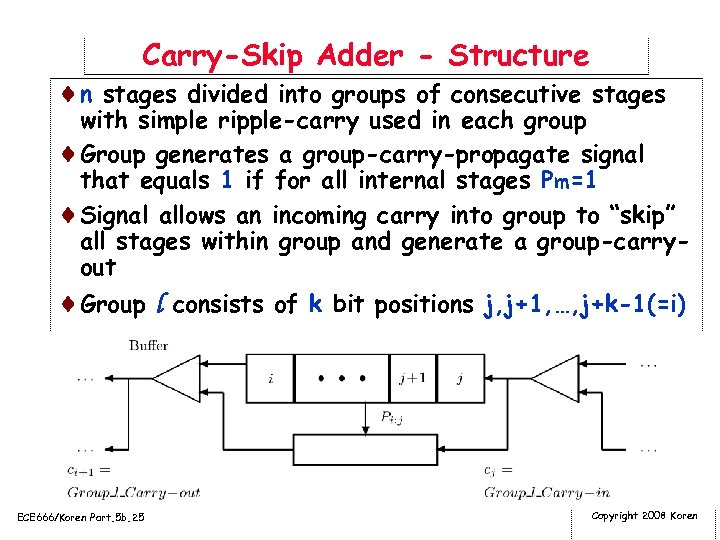Carry-Skip Adder - Structure ¨n stages divided into groups of consecutive stages with simple ripple-carry used in each group ¨Group generates a group-carry-propagate signal that equals 1 if for all internal stages Pm=1 ¨Signal allows an incoming carry into group to “skip” all stages within group and generate a group-carryout ¨Group l consists of k bit positions j, j+1, …, j+k-1(=i) ECE 666/Koren Part. 5 b. 25 Copyright 2008 Koren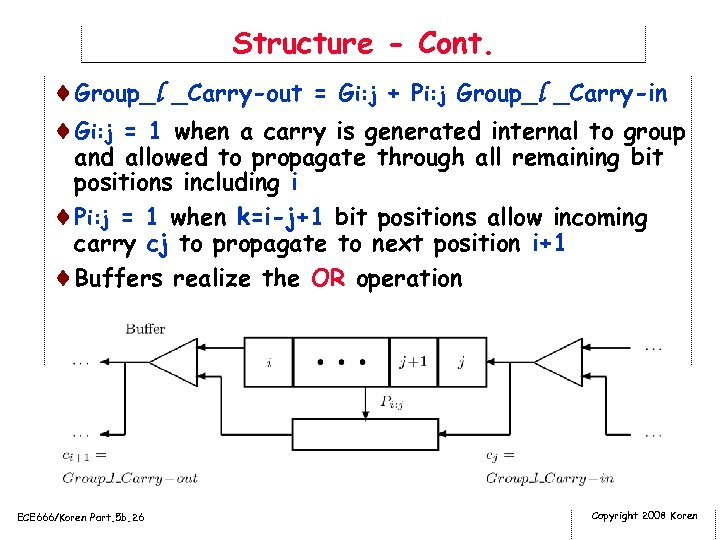Structure - Cont. ¨Group_l _Carry-out = Gi: j + Pi: j Group_l _Carry-in ¨Gi: j = 1 when a carry is generated internal to group and allowed to propagate through all remaining bit positions including i ¨Pi: j = 1 when k=i-j+1 bit positions allow incoming carry cj to propagate to next position i+1 ¨Buffers realize the OR operation ECE 666/Koren Part. 5 b. 26 Copyright 2008 Koren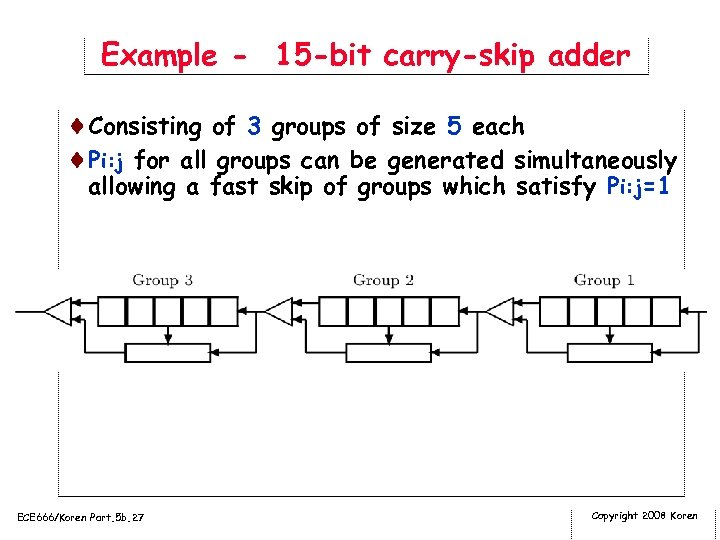Example - 15 -bit carry-skip adder ¨Consisting of 3 groups of size 5 each ¨Pi: j for all groups can be generated simultaneously allowing a fast skip of groups which satisfy Pi: j=1 ECE 666/Koren Part. 5 b. 27 Copyright 2008 Koren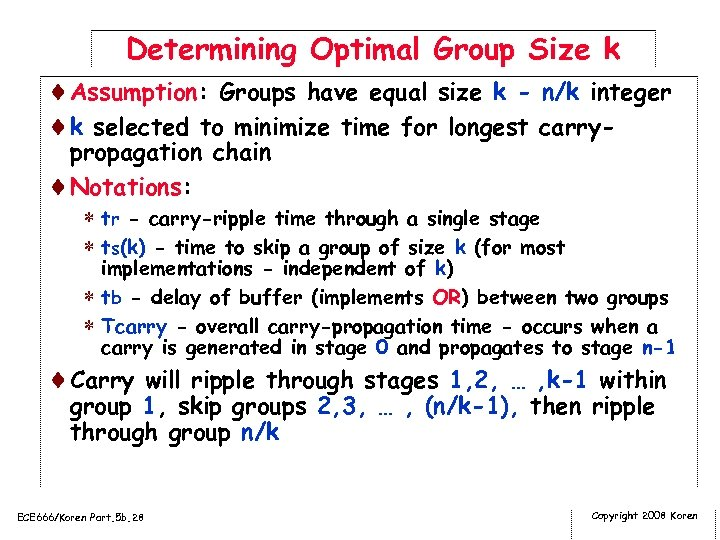Determining Optimal Group Size k ¨Assumption: Groups have equal size k - n/k integer ¨k selected to minimize time for longest carrypropagation chain ¨Notations: * tr - carry-ripple time through a single stage * ts(k) - time to skip a group of size k (for most implementations - independent of k) * tb - delay of buffer (implements OR) between two groups * Tcarry - overall carry-propagation time - occurs when a carry is generated in stage 0 and propagates to stage n-1 ¨Carry will ripple through stages 1, 2, … , k-1 within group 1, skip groups 2, 3, … , (n/k-1), then ripple through group n/k ECE 666/Koren Part. 5 b. 28 Copyright 2008 Koren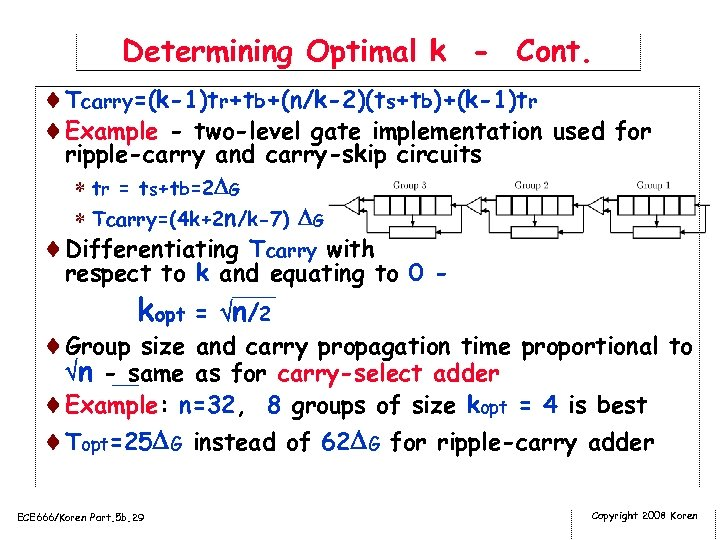Determining Optimal k - Cont. ¨Tcarry=(k-1)tr+tb+(n/k-2)(ts+tb)+(k-1)tr ¨Example - two-level gate implementation used for ripple-carry and carry-skip circuits * tr = ts+tb=2 G * Tcarry=(4 k+2 n/k-7) G ¨Differentiating Tcarry with respect to k and equating to 0 _____ ¨ kopt = n/2 ¨Group size and carry propagation time proportional to n -___ same as for carry-select adder ¨Example: n=32, 8 groups of size kopt = 4 is best ¨Topt=25 G instead of 62 G for ripple-carry adder ECE 666/Koren Part. 5 b. 29 Copyright 2008 Koren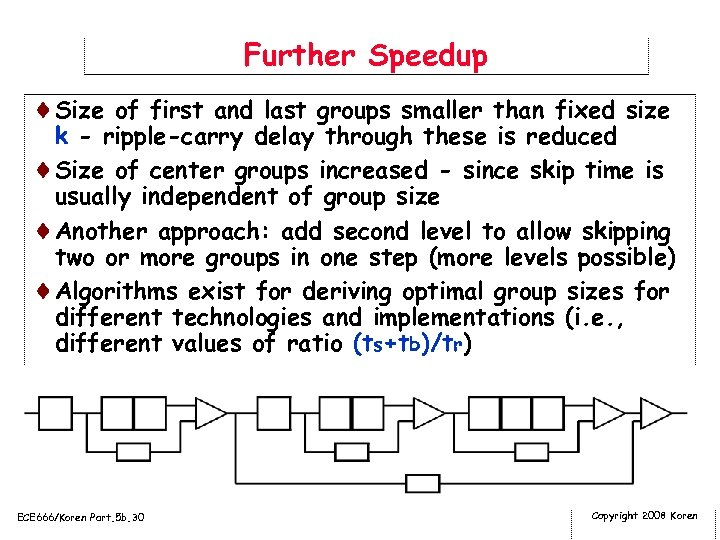Further Speedup ¨Size of first and last groups smaller than fixed size k - ripple-carry delay through these is reduced ¨Size of center groups increased - since skip time is usually independent of group size ¨Another approach: add second level to allow skipping two or more groups in one step (more levels possible) ¨Algorithms exist for deriving optimal group sizes for different technologies and implementations (i. e. , different values of ratio (ts+tb)/tr) ECE 666/Koren Part. 5 b. 30 Copyright 2008 Koren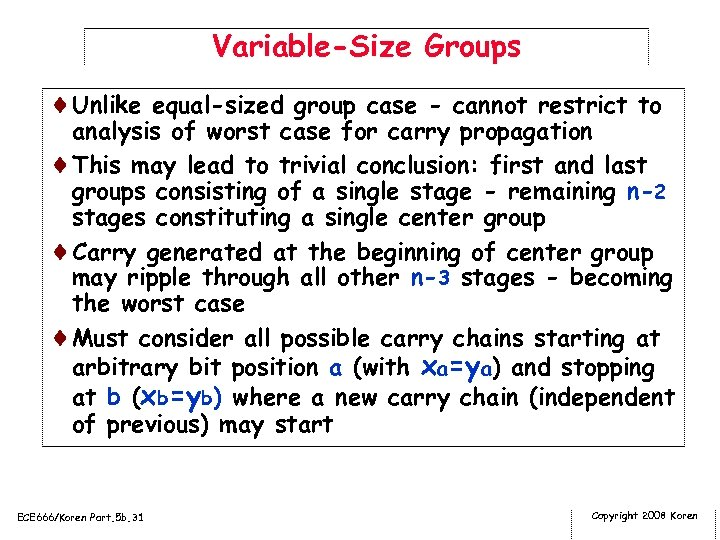Variable-Size Groups ¨Unlike equal-sized group case - cannot restrict to analysis of worst case for carry propagation ¨This may lead to trivial conclusion: first and last groups consisting of a single stage - remaining n-2 stages constituting a single center group ¨Carry generated at the beginning of center group may ripple through all other n-3 stages - becoming the worst case ¨Must consider all possible carry chains starting at arbitrary bit position a (with xa=ya) and stopping at b (xb=yb) where a new carry chain (independent of previous) may start ECE 666/Koren Part. 5 b. 31 Copyright 2008 Koren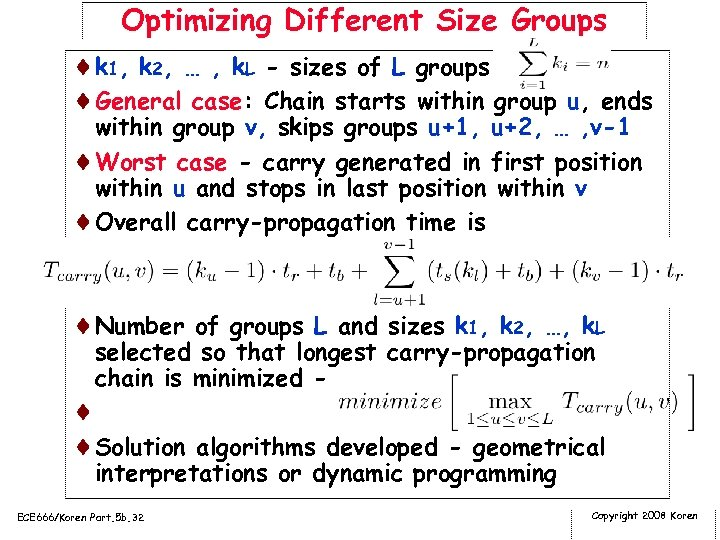Optimizing Different Size Groups ¨k 1, k 2, … , k. L - sizes of L groups ¨General case: Chain starts within group u, ends within group v, skips groups u+1, u+2, … , v-1 ¨Worst case - carry generated in first position within u and stops in last position within v ¨Overall carry-propagation time is ¨Number of groups L and sizes k 1, k 2, …, k. L selected so that longest carry-propagation chain is minimized - ¨ ¨Solution algorithms developed - geometrical interpretations or dynamic programming ECE 666/Koren Part. 5 b. 32 Copyright 2008 Koren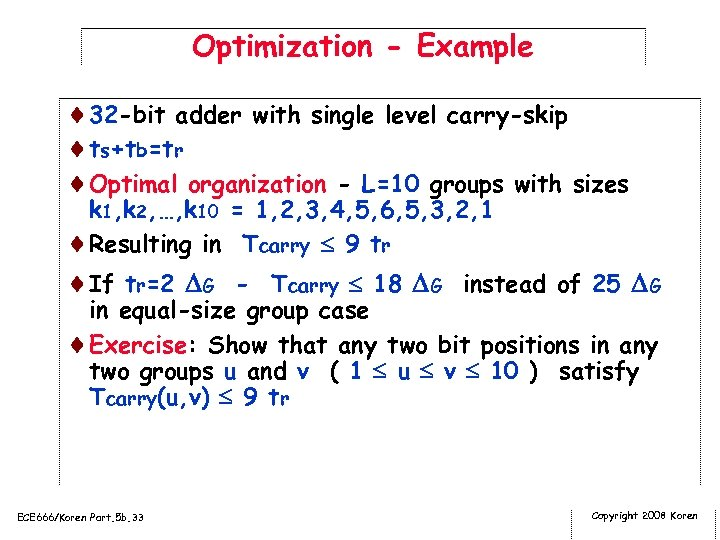Optimization - Example ¨ 32 -bit adder with single level carry-skip ¨ts+tb=tr ¨Optimal organization - L=10 groups with sizes k 1, k 2, …, k 10 = 1, 2, 3, 4, 5, 6, 5, 3, 2, 1 ¨Resulting in Tcarry 9 tr ¨If tr=2 G - Tcarry 18 G instead of 25 G in equal-size group case ¨Exercise: Show that any two bit positions in any two groups u and v ( 1 u v 10 ) satisfy Tcarry(u, v) 9 tr ECE 666/Koren Part. 5 b. 33 Copyright 2008 Koren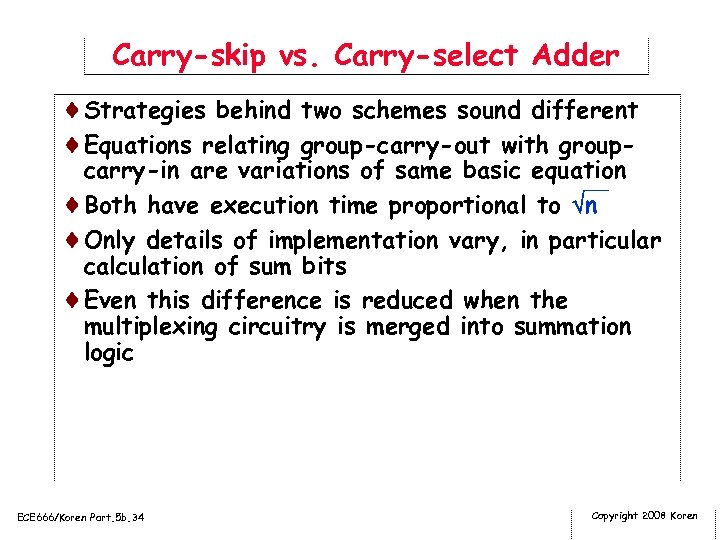Carry-skip vs. Carry-select Adder ¨Strategies behind two schemes sound different ¨Equations relating group-carry-out with group- carry-in are variations of same basic equation ___ ¨Both have execution time proportional to n ¨Only details of implementation vary, in particular calculation of sum bits ¨Even this difference is reduced when the multiplexing circuitry is merged into summation logic ECE 666/Koren Part. 5 b. 34 Copyright 2008 Koren